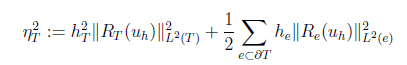# Local error estimation on the element boundary

Hi,

I am trying to apply the Dorfler marking criterion for an adaptive mesh, which uses the following eta:Here, the second term R_e is proportional to the jump in the normal derivative of u_h across the edge e shared by this element and a neighboring element. And I am having trouble calculating it. First, as I checked the example codes for mesh adaptivity, I found only

``````elerr = Integrate (err, mesh, VOL, element_wise=True)
``````

which is an element-wise integration on the whole element.

I have two questions:

1. Is there any way to do element-wise integration on the boundary of each element? I saw someone calculating similar things using `dx(element_boundary=True)`, what is the difference between this and `ds(element_boundary=True)`?
2. When we generate the normal vectors by `n = specialcf.normal(2)`
How is its direction decided when I evaluate it on an interior edge?